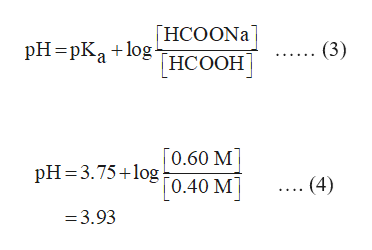# A buffer is prepared by adding 0.40 moles of formic acid (HCOOH) and 0.60 moles of sodium formate (HCOONa) to enough water to make 1.00 L of solution.a. Calculate the pH of this buffer.b. How many moles of HCOONa must be added to 1.0 L of a 0.40M HCOOH solution to prepare a buffer with pH 4.24?c. Calculate the change in pH for 100mL of the buffers from part a) and b) upon the addition of 5.00 mL of 1.00M HCl.

Question
33 views

A buffer is prepared by adding 0.40 moles of formic acid (HCOOH) and 0.60 moles of sodium formate (HCOONa) to enough water to make 1.00 L of solution.

a. Calculate the pH of this buffer.

b. How many moles of HCOONa must be added to 1.0 L of a 0.40M HCOOH solution to prepare a buffer with pH 4.24?

c. Calculate the change in pH for 100mL of the buffers from part a) and b) upon the addition of 5.00 mL of 1.00M HCl.

check_circle

Step 1

A solution which resists the change in pH when a small amount of base or acid is added to it is known as a buffer solution. The behaviour of a buffer is described by the Henderson-Hasselbalch equation. The Henderson-Hasselbalch equation for a mixture of weak acids and its salts with a strong base is shown below:

Step 2

The standard Ka of HCOOH is 1.77×10-4. The pKa is the negative logarithm of the dissociation constant of the weak acid which is expressed by equation (1).

Substitute, 1.77×10-4 in equation (1) as shown in equation (2).

Step 3

(a)

Calculate the resultant pH of the solution using the Henderson-Hasselbalch equation.The Henderson-Hasselbalch equation for the given reaction is expressed...help_outlineImage Transcriptionclose[HCOONA НСООН pH=pKa + log (3) [0.60 M] [0.40 M] pH=3.75+log .. (4) = 3.93 fullscreen

### Want to see the full answer?

See Solution

#### Want to see this answer and more?

Solutions are written by subject experts who are available 24/7. Questions are typically answered within 1 hour.*

See Solution
*Response times may vary by subject and question.
Tagged in

### Chemistry